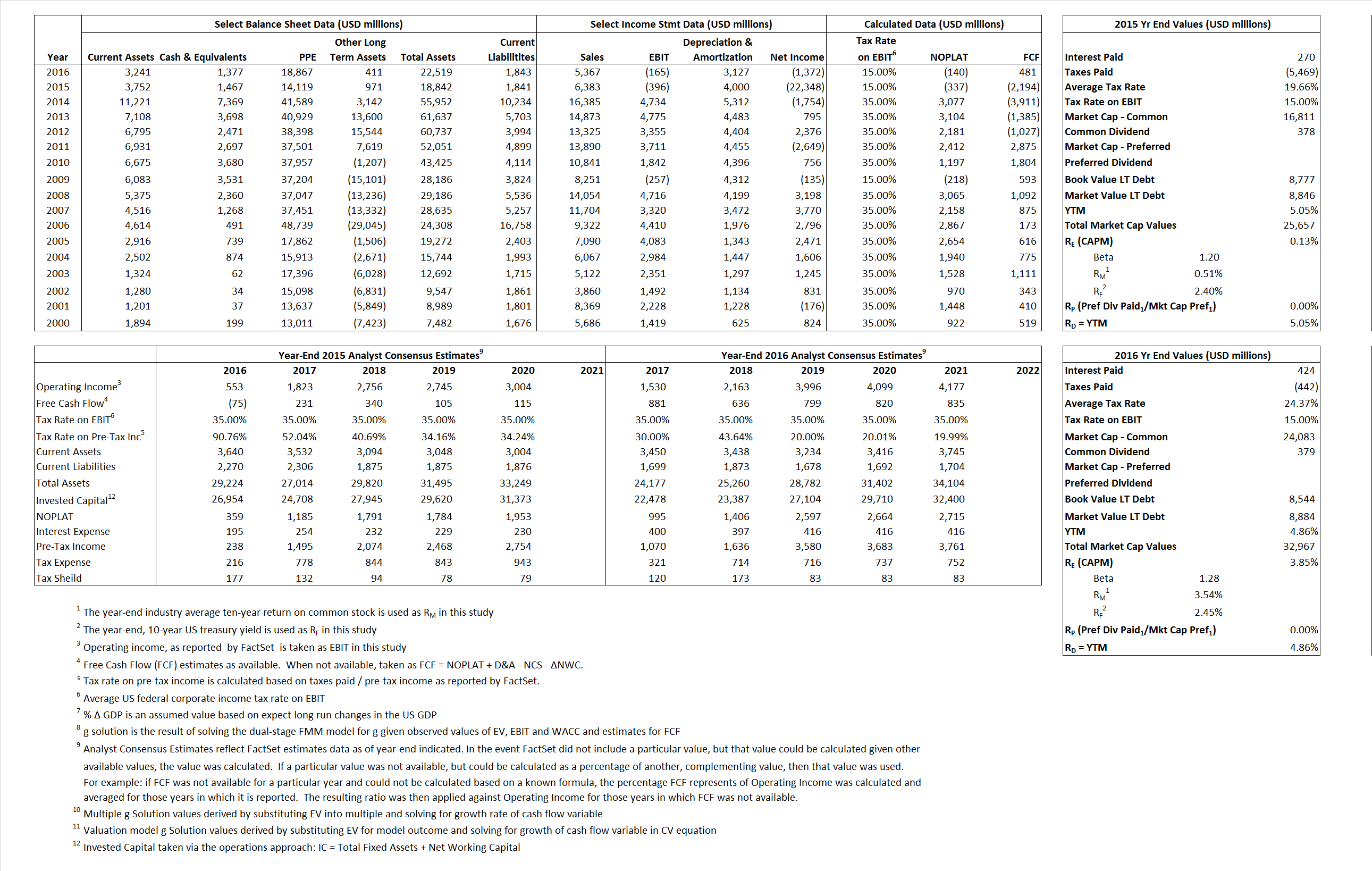# Apache Corp.

## Analyst Listing

The following analysts provide coverage for the subject firm as of May 2016:

 Broker Analyst Analyst Email Wells Fargo Securities David Tameron david.tameron@wellsfargo.com BMO Capital Markets Phillip Jungwirth phillip.jungwirth@bmo.com Johnson Rice & Company Charles Meade cmeade@jrco.com Evercore ISI Stephen Richardson stephen.richardson@evercoreisi.com Scotia Howard Weil Peter Kissel peter.kissel@scotiabank.com Raymond James John Freeman john.freeman@raymondjames.com Deutsche Bank Research Ryan Todd ryan.todd@db.com Wolfe Research Paul Sankey psankey@wolferesearch.com RBC Capital Markets Scott Hanold scott.hanold@rbccm.com Atlantic Equities Barry MacCarthy b.maccarthy@atlantic-equities.com Capital One Securities Richard Tullis richard.tullis@capitalone.com Credit Suisse Edward Westlake edward.westlake@credit-suisse.com Piper Jaffray Pearce Hammond pearce.w.hammond@simmonspjc.com Alembic Global Advisors James Sullivan james.sullivan@alembicglobal.com IBERIA Capital Partners Eric Fox eric.fox@iberiabank.com Cowen & Company Charles Robertson charles.robertson@cowen.com Jefferies Jonathan D. Wolff jwolff@jefferies.com Nomura Research Lloyd Byrne lloyd.byrne@nomura.com Seaport Global Securities Michael Kelly mkelly@seaportglobal.com KLR Group Gail Nicholson gn@klrgroup.com

## Primary Input Data## Derived Input Data

### Equational Form

Net Operating Profit Less Adjusted Taxes NOPLAT  (337) (140)$NOPLAT\, =\, EBIT\, x\, (1 \,-\, Avg \,\,Tax\,\, Rate\,\, on\,\, EBIT)$
Free Cash Flow FCF (2,194) 481$FCF\,=NOPLAT\,+\,Non-Cash\,Expenses-\Delta NWC\,-\,NCS$
Tax Shield TS 53 103$TS\,=\,Interest\,\,Paid\,\,x\,\, Avg \,\,Tax\,\,Rate\,\, on\,\, Pre-Tax\,\, Income$
Invested Capital IC 17,001 20,676$IC\,=\,Fixed\,\,Operating\,\,Assets\,\,+\,\,Net\,\, Working\,\, Capital$
Return on Invested Capital ROIC -1.98% -0.68%$ROIC\,=\,\frac { NOPLAT }{ IC }$
Net Investment NetInv (24,717) 6,802$NetInv\,=\,{ {IC}_{1}}-{{IC}_{0}}+Depreciation$
Investment Rate IR  7,343.14% -4,849.91%$IR\,=\,\frac {NetInv}{NOPLAT}$
Weighted Average Cost of Capital
WACCMarket 1.49% 3.80%$WACC\,=\,\frac { E }{ V } { R }_{ E }\,+\,\frac { P }{ V } { R }_{ P }\,+\,\frac { D }{ V } { R }_{ D }\left( 1- Avg\,\, Tax\,\,Rate\,\,on\,\,Pre-Tax\,\,Income \right)$
WACCBook     8.20%     8.88%
Enterprise value
EVMarket  24,190 31,590$EV\,=\,Market\,\,Cap\,\,Equity\,+\,\,Long\,\,Term\,\,Debt\,-\,Cash$
EVBook     24,121     31,250
Long-Run Growth
g = IR x ROIC
-145.39%     32.90% Long-run growth rates of the income variable are used in the Continuing Value portion of the valuation models.
g = %$\Delta$ GDP     2.50%     2.50%
Margin from Operations M    -6.20%    -3.07%$M\,\,=\,\,\frac{EBIT}{SALES}$
Depreciation/Amortization Rate D   110.99%    105.57%$D\,\,=\,\,\frac{D+A}{EBITDA}$

## Valuation Multiple Outcomes

The outcomes presented in this study are the result of original input data, derived data, and synthesized inputs.

### model g solution

12/31/2015 12/31/2016 12/31/2015 12/31/2016 12/31/2015 12/31/2016

EV/SALES$\frac {EV}{Sales} \,= \,\frac{ROIC\, -\, g}{ROIC\,(WACC\,-\,g)}\,(1\,-\,T)\,(M)$

3.79  5.89  9.68%  12.28%  8.58%  9.12%

EV/EBITDA$\frac {EV}{EBITDA} \,= \,\frac{ROIC\, -\, g}{ROIC\,(WACC\,-\,g)}\,(1\,-\,T)\,(1\,-\,D)$

6.71 10.66 9.68% 12.28% 8.58% 9.12%

EV/NOPLAT$\frac {EV}{NOPLAT} \,= \,\frac{ROIC\, -\, g}{ROIC\,(WACC\,-\,g)}$

-71.87 -225.24 9.68% 12.28% 8.58% 9.12%

EV/FCFOPS$\frac {EV}{FCF_{OPS}} \,= \,\frac{ROIC\, -\, g}{ROIC\,(WACC\,-\,g)}\,(1\,-\,T)$

-11.03  65.67 9.68% 12.28% 8.58% 9.12%

EV/EBIT$\frac {EV}{EBIT} \,= \,\frac{ROIC\, -\, g}{ROIC\,(WACC\,-\,g)}\,(1\,-\,T)$

-61.09 -191.45 9.68% 12.28% 8.58% 9.12%

EV/IC$\frac {EV}{IC} \,= \,\frac{ROIC\, -\, g}{WACC\,-\,g}$

1.42 1.53 9.68% 12.28% 8.58% 9.12%